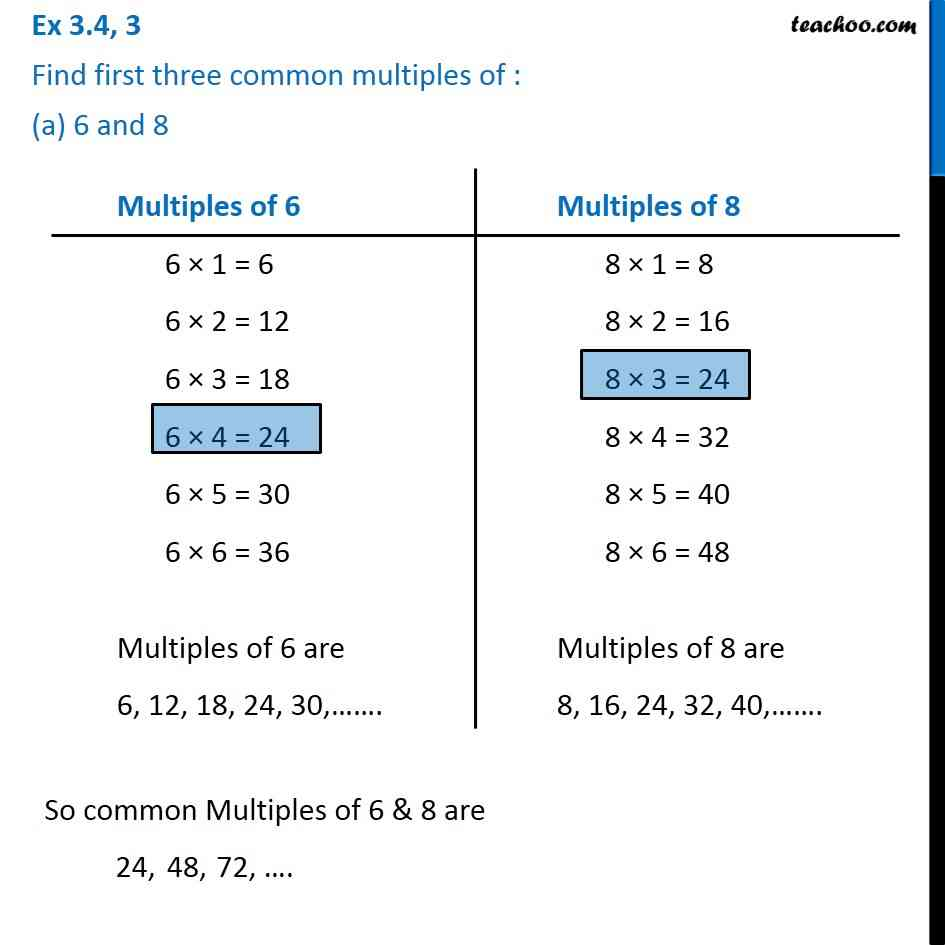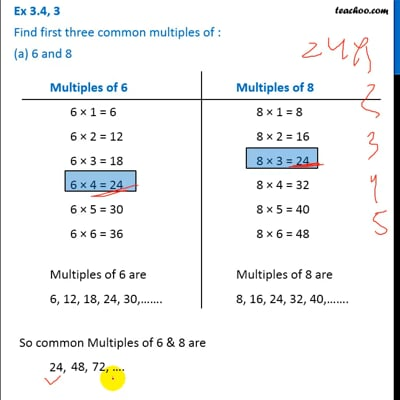Ex 3.4

Chapter 3 Class 6 Playing with Numbers
Serial order wiseThis video is only available for Teachoo black users

Introducing your new favourite teacher - Teachoo Black, at only ₹83 per month

### Transcript

Ex 3.4, 3 Find first three common multiples of : (a) 6 and 8Multiples of 6 6 × 1 = 6 6 × 2 = 12 6 × 3 = 18 6 × 4 = 24 6 × 5 = 30 6 × 6 = 36 Multiples of 6 are 6, 12, 18, 24, 30,……. Multiples of 8 8 × 1 = 8 8 × 2 = 16 8 × 3 = 24 8 × 4 = 32 8 × 5 = 40 8 × 6 = 48 Multiples of 8 are 8, 16, 24, 32, 40,……. So common Multiples of 6 & 8 are 24,